The OPTEX Procedure

### Design Coding

The way the independent effects of the model are interpreted to generate a linear model is called coding. The OPTEX procedure provides for different types of coding. For D-optimality, the type of coding affects only the absolute value of the computed efficiency criteria, not the relative values for two different designs. Thus, different codings do not affect the choice of D-optimal design. In this section, the details and ramifications of the different types of coding are discussed.

Coding the points in a design involves selecting linearly independent columns corresponding to each model term, turning particular values of the factors into a row vector. The OPTEX procedure requires a nonsingular coding for the design matrix. Because of this, any two coding schemes are related by a nonsingular transformation.

#### Static Coding

The default coding for the design points is as follows:

• Unless you specify CODING=NONE (or NOCODE) in the PROC OPTEX statement, continuous variables are centered and scaled so that their maximum and minimum values are 1 and –1, respectively.

• The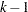columns corresponding to the main effect of a classification variable A are computed as follows: For a design point with A at itsth level, for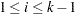, the columns of the design matrix associated with A are all 0 except for theth column, which is 1. When A is at itsth level, allcolumns associated with A are
. Thus, if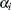denotes the expected response at theth level of A, thecolumns yield estimates of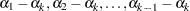.

• Columns for crossed effects are computed by taking the horizontal direct product of columns corresponding to the constituent effects.

This coding corresponds to modeling without over-parameterization, by using the same method as the CATMOD procedure in SAS/STAT® software. This is different from the method used by the GLM procedure, which uses an over-parameterized model.

#### Orthogonal Coding

If you specify CODING=ORTH or CODING=ORTHCAN, the points are first coded as described in the previous section and then recoded so that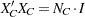, where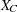is the design matrix for the candidate points,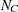is the number of candidates, andis the identity matrix. This is required in order for the D- and A-efficiency measures to make sense. For the option CODING=ORTHCAN, this recoding is accomplished by computing a square matrix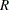such that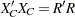and then transforming each row vectoras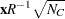If you specify CODING=ORTH, the recoding is done in a similar fashion, except that the matrixis computed according to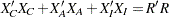, where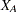and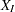are the design matrices for the AUGMENT= and INITDESIGN= data sets, respectively (coded as described in the previous section.) Thus, these two orthogonal coding options only differ when there is an AUGMENT= or an INITDESIGN= data set ; the option CODING=ORTH includes points from these data sets in computing the orthogonal coding, while the option CODING=ORTHCAN uses only the candidates themselves.

#### Example of Coding

For example, consider a main effect model with one continuous variable X and one three-level classification variable A. The results of the various coding options are shown in Table 10.7.

Table 10.7 Different Types of Design Coding

Original

Design Matrix With

Design Matrix With

Design Matrix With

Data

CODING=NONE

CODING=STATIC

CODING=ORTH

X

A

X

A1

A2

X

A1

A2

X

A1

A2

1

1

1

1

1

0

1

–1

1

0

1

–1.464

0.598

–0.707

2

2

1

2

0

1

1

–0.6

0

1

1

–0.878

–0.478

1.414

3

3

1

3

–1

–1

1

–0.2

–1

–1

1

–0.293

–1.554

–0.707

4

1

1

4

1

0

1

0.2

1

0

1

0.293

1.554

–0.707

5

2

1

5

0

1

1

0.6

0

1

1

0.878

0.478

1.414

6

3

1

6

–1

–1

1

1

–1

–1

1

1.464

–0.598

–0.707

The first column in each design matrix is an all-ones vector corresponding to the intercept, the next column corresponds to the linear effect of X, and the last two columns correspond to the two degrees of freedom for the main effect of A.

#### General Recommendations

Coding does not affect the relative ordering of designs by D-efficiency, and the same is true for G-efficiency and the average standard error of prediction. This is easy to see for the latter two measures, which are based on the variance of prediction, since how accurately a point is predicted should not be affected by how the independent variables are coded. For D-optimality, note again that coding corresponds to multiplying the design matrix on the right by some nonsingular transformation A, which changes the determinant of the information matrix as follows: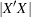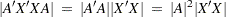Thus, recoding simply multiplies the D-criterion by a constant that is the same for all designs. Note, however, that A-optimality is not invariant to coding.

Orthogonal coding will usually be the right one; it is not the default because it depends on the candidate set. Note, however, that for the distance-based criteria, if the distance between two points should be computed in terms of the actual values of the model variables instead of centered and scaled values, then you should specify CODING=NONE or NOCODE. The NOCODE option can also be useful when the NOINT option is specified.Previous Page | Next Page | Top of Page